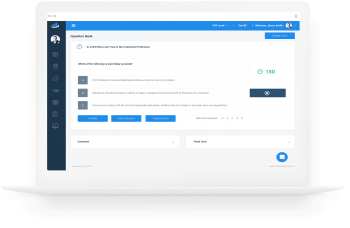Limited Time Offer: Save 10% on all 2021 and 2022 Premium Study Packages with promo code: BLOG10    Select your Premium Package »

Delta Hedging

Delta hedging involves adding up the deltas of the individual assets and options that make up a portfolio. A delta hedged portfolio is one for which the weighted sums of deltas of individual assets are zero. A position with a…

Option Greeks

The Greeks are a group of mathematical derivatives applied to help manage or understand portfolio risks. They include delta, gamma, theta, vega, and rho. Delta Delta is the rate of change of the option’s price attributable to a given change…

Black Model Valuation of Interest Rate Options and Swaptions

Interest Rate Options The underlying instrument in an interest rate swap is a reference interest rate. Reference rates include the Fed funds rate, Libor, and the rate on benchmark US Treasuries. Interest rate options are, therefore, options on forward rate…

Black Option Valuation Model

The black option valuation model is a modified version of the BSM model used for options on underlying securities that are costless to carry, including options on futures and forward contracts. Similar to the BSM model, the black model assumes…

Valuation of Equities and Currencies Using the Black-Scholes Model

Some underlying instruments have carry benefits. These benefits include dividends for stock options, foreign interest rates for currency options, and coupon payments for bond options. The BSM model should be adjusted to incorporate carry benefits in the option value. Let…

Components of the BSM Model

The BSM model for pricing options on a non-dividend-paying stock is given by: European Call $$c_0= S_0N(d_1) – e^{(-rT)}KN(d_2)$$ European Put $$p_0=e^{-rT}KN\left({-d}_2\right)-S_0N\left({-d}_1\right)$$ Where: \begin{align*} d_1 &=\frac{ln{\left(\frac{S}{K}\right)}+\left(r+\frac{1}{2}\sigma^2\right)T}{\sigma\sqrt T} \\ d_2 &=d_1-\sigma\sqrt T \end{align*} N(x) =…

Assumptions of the Black-Scholes-Merton Option Valuation Model

The Black-Scholes-Merton (BSM) model is an optional pricing model. Under this model, the underlying share prices evolve in continuous time and are characterized at any point in time by a continuous distribution rather than a discrete distribution. The following key…

Expectations Valuation Approach

One-step Binomial Tree Since a hedged portfolio returns the risk-free rate, it can determine the initial value of a call or put. The expectations approach calculates the values of the option by taking the present value of the expected terminal…

Interest rate options are options with an interest rate as the underlying. A call option on interest rates has a positive payoff when the current spot rate is greater than the exercise rate. \begin{align*} \text{Call option payoff} & =\text{Notional… Arbitrage Opportunities Involving Options Call Option A hedging portfolio can be created by going long $$\phi$$ units of the underlying asset and going short the call option such that the portfolio has an initial value of: V_0=\phi S_0-c_0  Where: $$S_0$$=The current stock…

Preparation Platform for the Three Levels of the CFA® ExamView More
Recent Posts
FRM® Study PlatformView More
Video Series – Level I of the CFA® Exam